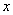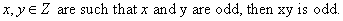Monday 08th August 2022CBSE Guess > Papers > Important Questions > Class XI > 2010 > Maths > Maths By Mr. Anil Kumar Tondak CBSE CLASS XI
Mathematical Reasoning Q.1. Write the negation of the following statements: (i) The number 2 is greater than 7. (ii) All triangles are not equilateral triangle. (iii) Australia is a continent. (iv) There does not exist a quadrilateral which has all its sides equal. (v) Every natural number is greater than 0. (vi) The sum of 3 and 4 is 9. Q.2. Find the component statements of the following compound statements and check whether they are true or false. (i) Number 3 is prime or it is odd. (ii) All integers are positive or negative. (iii) 100 is divisible by 3, 11 and 5. Q.3. State whether the “Or” used in the following statements is “exclusive “or” inclusive. Give reasons for your answer. (i) Sun rises or Moon sets. (ii) To apply for a driving licence, you should have a ration card or a passport. (iii) All integers are positive or negative. Q.4. Write the contrapositive of the following statements : (i) If a triangle is equilateral, it is isosceles. (ii) Ifis a prime number, thenis odd. Q.5. Write the converse of the following statements. (i) If a number n is even, then n2 is even. (ii) If you do all the exercises in the book, you get an A grade in the class. (iii) If two integers a and b are such that a > b, then a – b is always a positive integer. Q. 6. Check whether the following statement is true or not. IfQ.7. Using the words “necessary and sufficient” rewrite the statement “the integer n is odd if and only if n2 is odd”. Also check whether the statement is true. Q.8. Rewrite the following statements in the form of “p if and only if q”. (i) p : If you watch television, then you mind is free and if your mind is free, then you watch television. (ii) q : For you to get an A grade, it is necessary and sufficient that you do all the homework regularly Paper By Mr. Anil Kumar Tondak Email Id : [email protected] Ph No.: 9811363962